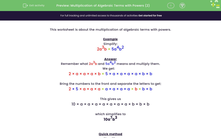# Multiply Algebraic Terms with Powers

In this worksheet, students will multiply more complicated algebraic terms.Key stage:  KS 3

Curriculum topic:   Algebra

Curriculum subtopic:   Simplify Algebraic Expressions to Maintain Equivalence

Popular topics:   Algebra worksheets

Difficulty level:#### Worksheet Overview

This activity is about the multiplication of algebraic terms with powers.

Example

Simplify:

2a3b × 5a4b2

Break down what 2a3b and 5a4b2 mean and multiply them.

We get:

2 × a × a × a × b × 5 × a × a × a × a × b × b

Bring the numbers to the front and separate the letters to get:

2 × 5 × a × a × a × a × a × a × a × b × b × b

This gives us

10 × a × a × a × a × a × a × a × b × b × b

which simplifies to

10a7b3

Quick method

Simplify:

2a3b × 5a4b2

Take the terms one at a time.

Start by multiplying the numbers (2 × 5 = 10).

Then deal with the indices of identical letters by adding the powers:  for a: 3 + 4 = 7 and for b: 1 + 2 = 3

Shall we try some questions now?

### What is EdPlace?

We're your National Curriculum aligned online education content provider helping each child succeed in English, maths and science from year 1 to GCSE. With an EdPlace account you’ll be able to track and measure progress, helping each child achieve their best. We build confidence and attainment by personalising each child’s learning at a level that suits them.

Get started